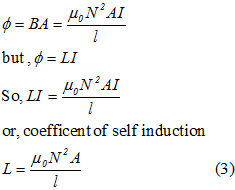# Self Inductance

## Self Inductance

• Consider the figure given below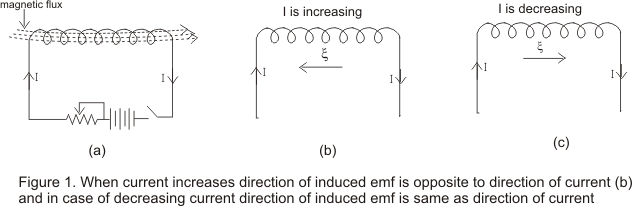• When we establish a current through an inductor or coil, it generates a magnetic field and this result in a magnetic flux passing through the coil as shown in figure 1(a).
• If we vary the amount of current flowing in the coil with time, the magnetic flux associated with the coil also changes and an emf ξ is induced in the coil.
• According to the Lenz's law, the direction of induced emf is such that it opposes its cause i.e. it opposes the change in current or magnetic flux.
• This phenomenon of production of opposing induced emf in inductor or coil itself due to time varying current in the coil is known as self induction.
• If I is the amount of current flowing in the coil at any instant then emf induced in the coil is directly proportional to the change in current i.e.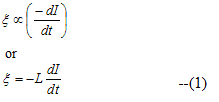where L is a constant known as coefficient of self induction.
• If (-dI/dt)=1 then ξ=L
Hence the coefficient of self induction of a inductor or coil is numerically equal to the emf induced in the coil when rate of change of current in the coil is unity.
• Now from the faraday's and Lenz's laws induced emf is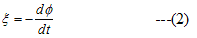comparing equation 1 and 2 we have,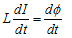or Φ=LI
• Again for I=1, Φ=L
hence the coefficient of self induction of coil is also numerically equal to the magnetic flux linked with the inductor carrying a current of one ampere
• If the coil has N number of turns then total flux through the coil is
Φtot=NΦ
where Φ is the flux through single turn of the coil .So we have,
Φtot=LI
or L=NΦ/I
for a coil of N turns
• In the figure given below consider the inductor to be the part of a circuit and current flowing in the inductor from left to right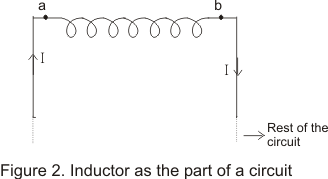• Now when a inductor is used in a circuit, we can use Kirchhoff�s loop rule and this emf(Self induced emf) can be treated as if it is a potential drop with point A at higher potential and B at lower potential when current flows from a to b as shown in the figure
• We thus have
Vab=LdI/dt

## Self induction of a long solenoid

• Consider a long solenoid of length l, area of cross-section A and having N closely wound turns.
• If I is the amount of current flowing through the solenoid them magnetic field B inside the solenoid is given by,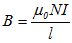• Magnetic flux through each turn of the solenoid is,